Question

# An aluminium object with a mass of 5.77 kg and at a temperature of 23.3 °C...

An aluminium object with a mass of 5.77 kg and at a temperature of 23.3 °C comes to thermal contact with a 9.26 kg copper object which is initially at a temperature of 97.7 °C. What is going to be the equilibrum temperature of the two objects? Neglect heat transfer between the objects and the environment. The specific heats are: cAl = 900 J/kg°C and cCu = 387 J/kg°C.

Dear student,

Find this solution.if any issue with that don't forget to write in comment section.I will rectify them as soon as possible.

If you find the solution helpful and kindly RATE THE ANSWER it would be appreciated.

Your rating is important to me.

Thanks for asking..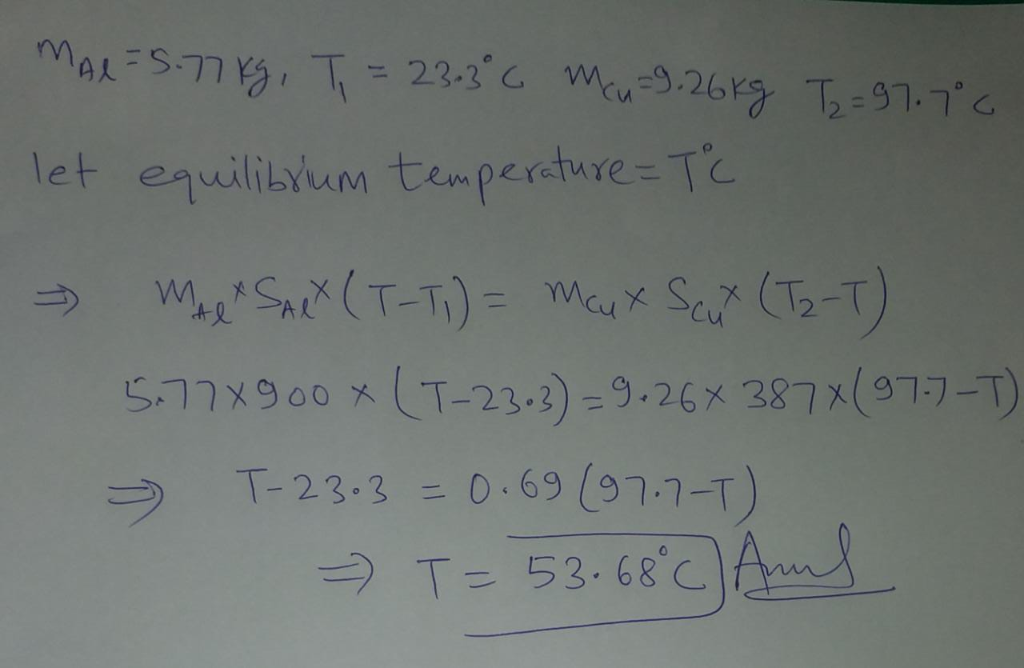#### Earn Coins

Coins can be redeemed for fabulous gifts.

Similar Homework Help Questions
• ### 3. A 100 g block of copper initially at 60°C is placed in contact with a...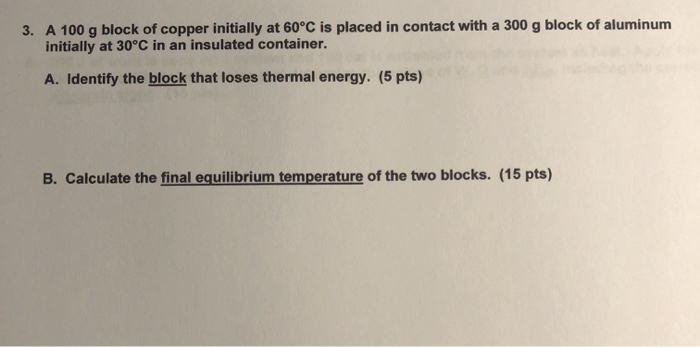3. A 100 g block of copper initially at 60°C is placed in contact with a 300 g block of aluminum initially at 30°C in an insulated container. A. Identify the block that loses thermal energy. (5 pts) B. Calculate the final equilibrium temperature of the two blocks. (15 pts) Copper a(Cu) = 17 x 10-61°C Ccu = 0.0923 cal/g.K = 386 J/kg-K LF (Cu) = 207 kJ/kg

• ### To determine the specific heat of an object, a student heats it to 100 ∘C in...

To determine the specific heat of an object, a student heats it to 100 ∘C in boiling water. She then places the 87.2 g object in a 173 galuminum calorimeter containing 136 g of water. The aluminum and water are initially at a temperature of 19.8 ∘, and are thermally insulated from their surroundings. If the final temperature is 23.4 ∘, what is the specific heat of the object? Referring to the table, identify the material in the object. Substance...

• ### SETR To determine the specific heat of an object, a student heats it to 100 C...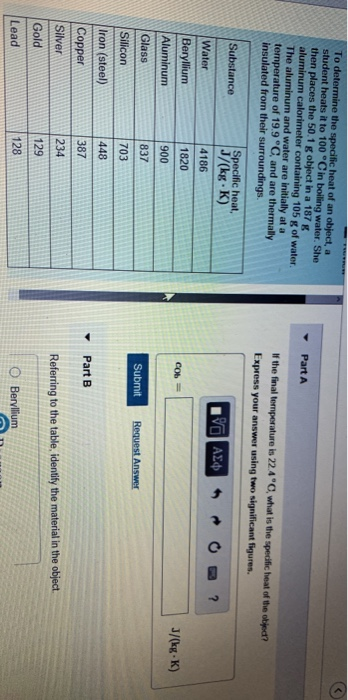SETR To determine the specific heat of an object, a student heats it to 100 C in boiling water. She then places the 50.1 g object in a 187 g aluminum calorimeter containing 105 g of water. The aluminum and water are initially at a temperature of 19.9 °C, and are thermally insulated from their surroundings. Part A If the final temperature is 22.4 C, what is the specific heat of the objed? Express your answer using two significant figures....

• ### Case 2: A 0.780-kg silver pellet with a temperature of 85 oC is added to 0.150...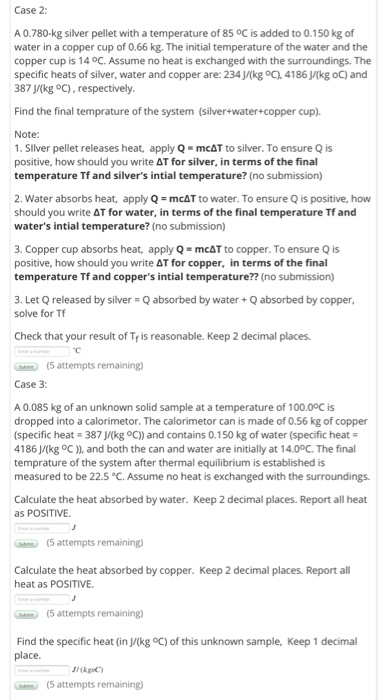Case 2: A 0.780-kg silver pellet with a temperature of 85 oC is added to 0.150 kg of water in a copper cup of 0.66 kg. The initial temperature of the water and the copper cup is 14 oc. Assume no heat is exchanged with the surroundings. The specific heats of silver, water and copper are: 234 J/(kg oC), 4186 J/(kg oC) and 387 J/(kg oc), respectively. Find the final temprature of the system (silver+water+copper cup). Note: 1. Slver pellet...

• ### The specific heat c is the amount of heat necessary to change the temperature of 1.00...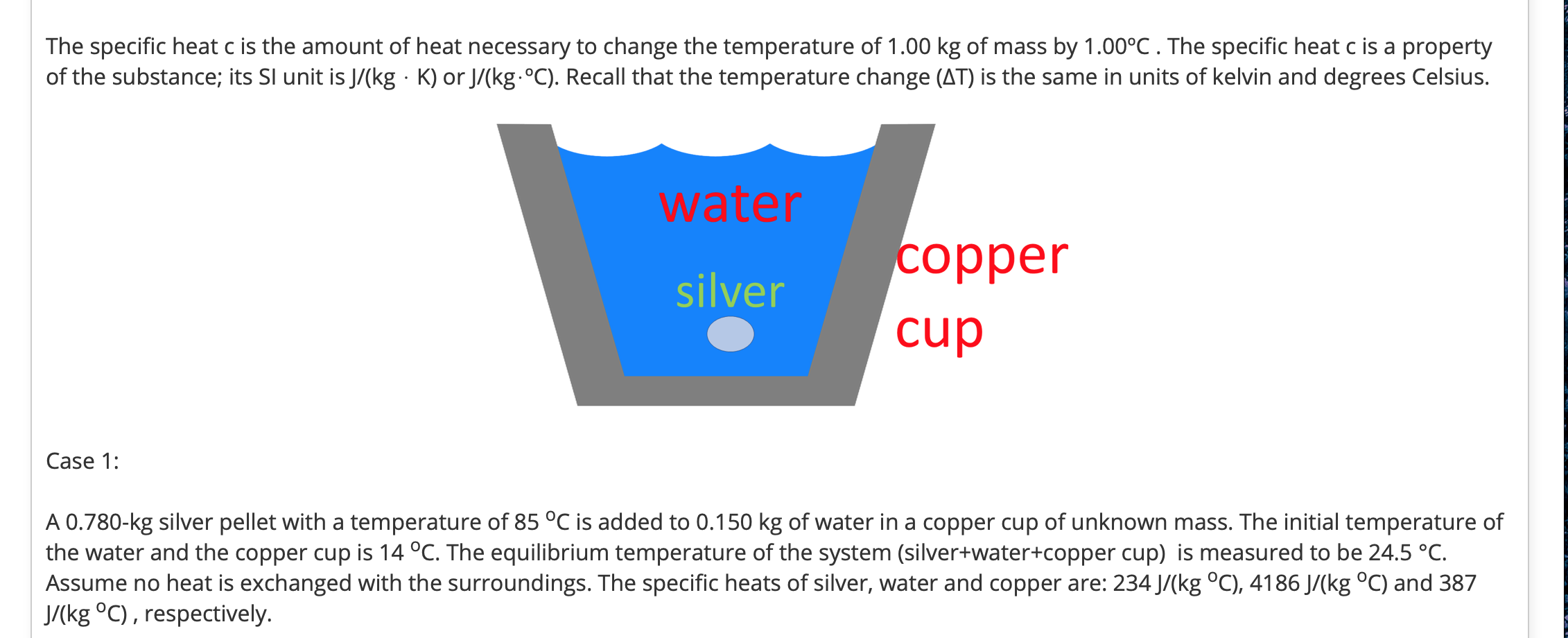The specific heat c is the amount of heat necessary to change the temperature of 1.00 kg of mass by 1.00°C. The specific heat c is a property of the substance; its Sl unit is J/(kg · K) or J/(kg.°C). Recall that the temperature change (AT) is the same in units of kelvin and degrees Celsius. water silver copper cup Case 1: A 0.780-kg silver pellet with a temperature of 85 °C is added to 0.150 kg of water in...

• ### 233 g of water at 16°C is contained in a copper container of mass 287 g....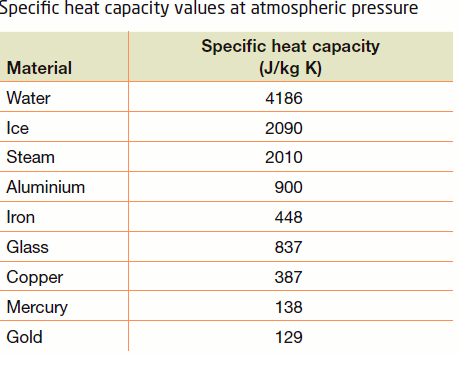233 g of water at 16°C is contained in a copper container of mass 287 g. An additional 134 g of water at 100°C is added. What is the final equilibrium temperature if we treat thesystem's water and container as isolated? Use the heat capacity values from the table Specific heat capacity values at atmospheric pressure Material Water lce Steam Aluminium Iron Glass Copper Mercury Gold Specific heat capacity (J/kg K) 4186 2090 2010 900 448 837 387 138 129

• ### please do Asap. 5. In the figure below, a magical red object has temperature 70°C and...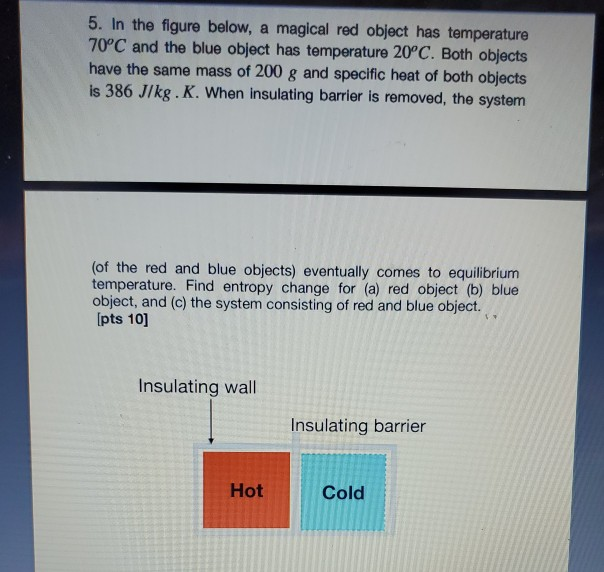please do Asap. 5. In the figure below, a magical red object has temperature 70°C and the blue object has temperature 20°C. Both objects have the same mass of 200 g and specific heat of both objects is 386 J/kg. K. When insulating barrier is removed, the system (of the red and blue objects) eventually comes to equilibrium temperature. Find entropy change for (a) red object (b) blue object, and (c) the system consisting of red and blue object. [pts...

• ### A 3.2 kg block of copper at a temperature of 82°C is dropped into a bucket...

A 3.2 kg block of copper at a temperature of 82°C is dropped into a bucket containing a mixture of ice and water whose total mass is 1.2 kg. When thermal equilibrium is reached the temperature of the water is 8°C. How much ice was in the bucket before the copper block was placed in it? (Neglect the heat capacity of the bucket.) answer in kg

• ### Two initially separated objects, make thermal contact, m_1, c_1 and T_1 are respectively the mass, specific...

Two initially separated objects, make thermal contact, m_1, c_1 and T_1 are respectively the mass, specific heat and temperature of the first object and m_2, c_2 and T_2 are respectively the corresponding of the second object. The initial temperatures are T_20 <T_10. While body 1 slowly cools, 2 heats up slowly. a) Calculate T_2 (T_1) b) Find the change in deltropy entropy as a function of T_1 c) show that deltaS is a maximum when both bodies reach thermal equilibrium

• ### - -/10 points Thermo: Calorimetry - ice + steam 0/6 Submissions Used My Ne 0.1 kg of steam at 100°C is added to 0....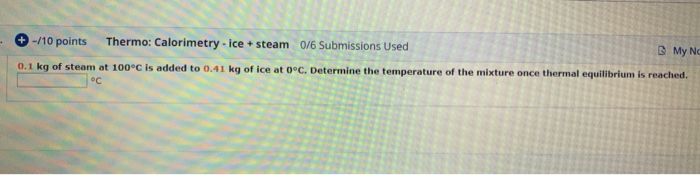- -/10 points Thermo: Calorimetry - ice + steam 0/6 Submissions Used My Ne 0.1 kg of steam at 100°C is added to 0.41 kg of ice at 0°C. Determine the temperature of the mixture once thermal equilibrium is reached. 8. -70 points Specific Heats & Latent Heats 070 Submissions Used These values will be needed for the following calorimetry problems. SPECIFIC HEATS Specific Heat Substance (J/kg.K) Aluminum 900 Copper 390 Glycerin 2410 2100 Iron 450 Lead 130 Mercury 140...